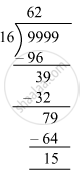# What least number must be added to 1056 to get a number exactly divisible by 23? - Mathematics

Fill in the Blanks

Find the largest 4-digit number divisible by 16.

#### Solution

We have to find the largest four digit number divisible by 16 .
The largest four-digit number = 9999
Therefore, dividend =9999
Divisor =16Here, we get remainder =15
Therefore, 15 must be subtracted from 9999 to get the largest four digit number that is divisible by 16.
i.e., 9999 − 15 = 9984
Thus, 9984 is the largest four-digit number that is divisible by 16.

Is there an error in this question or solution?

#### APPEARS IN

RS Aggarwal Class 6 Mathematics
Chapter 3 Whole Numbers
Exercise 3E | Q 10 | Page 56